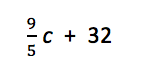### Program temperature.py

• Prompts user for a integer Celsius temperature and separately computes its integer and decimal Fahrenheit equivalent using the formula• Two different possible runs

Enter Celsius temperature: 12

12 C = 53 F

12 C = 53.6 F

Enter Celsius temperature: 34

34 C = 93 F

34 C = 93.2 F

### Program can_you_relate.py

• Prompts user for two numbers and computes their mathematical relationships
• Two different possible runs

Enter number: 12

Enter number: 14

12 < 14 : True

12 > 14 : False

12 <= 14 : True

12 >= 14 : False

12 == 14 : False

12 != 14 : True

Enter number: 8

Enter number: 8

8 < 8 : False

8 > 8 : False

8 <= 8 : True

8 >= 8 : True

8 == 8 : True

8 != 8 : False

### Program break_the_bank.py

• With a single prompt, it gets from the user four values in the following order: number of quarters, dimes, nickels, and pennies. Computes and displays how much the coinage is worth.
• Two different possible runs

Number of quarters, dimes, nickels, and pennies: 27 14 28 18

Coinage

27 quarters

14 dimes

28 nickels

18 pennies

are worth 9.73 dollars

Number of quarters, dimes, nickels, and pennies: 1 2 3 4

Coinage

1 quarters

2 dimes

3 nickels

4 pennies

are worth 0.6400000000000001 dollars

• For those who dislike Python's approximation in the second test run, might consider the built-in function `round()`. Function `round()` can take one or two arguments. The first argument is the value to be rounded, the second optional value is the wanted number of places after the decimal. When a second value is not supplied, it rounds to an integer.

### Program logical_operators.py

• Introduces the logical binary operators `and` and `or` and the unary logical operator `not`
• A program run

##### Logical values (bool)

True

False

##### Logical and

True and True = True

True and False = False

False and True = False

False and False = False

##### Logical or

True or True = True

True or False = True

False or True = True

False or False = False

##### Logical negation

not True = False

not False = True